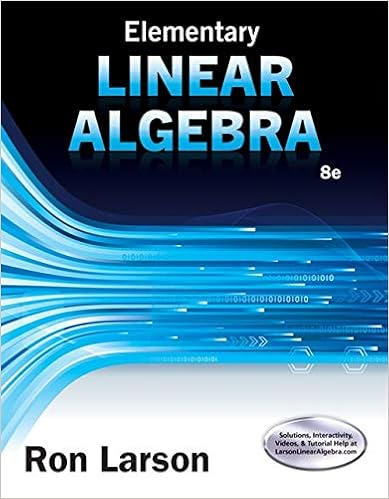# 18 actually this exercise could be a lemma for

• Notes
• 276

This preview shows page 253 - 256 out of 276 pages.

##### We have textbook solutions for you!
The document you are viewing contains questions related to this textbook.The document you are viewing contains questions related to this textbook.
Chapter 6 / Exercise 14
Elementary Linear Algebra
LarsonExpert Verified
18. Actually, this exercise could be a lemma for Exercise 7.2.17. (a) It is nilpotent by Exercise 7.2.12 since M is a upper triangular matrix with each diagonal entry equal to zero. (b) It comes from some direct computation. 252
##### We have textbook solutions for you!
The document you are viewing contains questions related to this textbook.The document you are viewing contains questions related to this textbook.
Chapter 6 / Exercise 14
Elementary Linear Algebra
LarsonExpert Verified
(c) Since MD = DM , we have J r = ( M + D ) r = r k = 0 r k M k D r - k . The second equality is due to the fact that M k = O for all k p . 19. (a) It comes from some direct computation. Multiplying N at right means moving all the columns to their right columns. (b) Use Exercise 7.2.18(c). Now the matrix M is the matrix N in Exercise 7.2.19(a). (c) If λ < 1, then the limit tends to a zero matrix. If λ = 1 amd m = 1, then the limit tends to the identity matrix of dimension 1. Con- versely, if λ 1 but λ 1, then the diagonal entries will not converge. If λ = 1 but m > 1, the 12-entry will diverge. (d) Observe the fact that if J is a Jordan form consisting of several Jordan blocks J i , then J r = i J r i . So the lim m J m exsist if and only if lim m J m i exists for all i . On the other hand, if A is a square matrix with complex entries, then it has the Jordan canonical form J = Q - 1 AQ for some Q . This means that lim m A m exists if and only if lim m J m exists. So Theorem 5.13 now comes from the result in Exercise 7.2.19(c). 20. (a) The norm A 0 since A ij 0 for all i and j . The norm A = 0 if and only if A ij = 0 for all i and j . So A = 0 if and only if A = O . (b) Compute cA = max { cA ij } = c max { A ij } = c A . (c) It comes from the fact A ij + B ij A ij + B ij for all i and j . (d) Compute AB = max { ( AB ) ij } = max { n k = 1 A ik B kj } max { n k = 1 A B } = n A B . 21. (a) The Corollary after 5.15 implies that A m is again a transition matrix. So all its entris are no greater than 1. 253
(b) Use the inequaliy in Exercise 7.2.20(d) and the previous argument. We compute J m = P - 1 A m P n 2 P - 1 A m P n 2 P - 1 P . Pick the fixed value c = n 2 P - 1 P and get the result. (c) By the previous argument, we know the norm J m is bounded. If J 1 is a block corresponding to the eigenvalue 1 and the size of J 1 is greater than 1, then the 12-entry of J m 1 is unbounded. This is a contradiction. (d) By the Corollary 3 after Theorem 5.16, the absolute value of eigen- values of A is no greater than 1. So by Theorem 5.13, the limit lim m A m exists if and only if 1 is the only eigenvalue of A . (e) Theorem 5.19 confirm that dim ( E 1 ) = 1. And Exercise 7.2.21(c) implies that K 1 = E 1 . So the multiplicity of the eigenvalue 1 is equal to dim ( K 1 ) = dim ( E 1 ) = 1 by Theorem Theorem 7.4(c).
•••